﻿

### A characterization of groups of exponent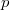$p$ which are nilpotent of class at most 2

#### Abstract

Let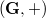$(\mathbf{G},+)$ be a group of prime exponent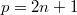$p = 2n + 1$. In this paper we prove that$(\mathbf{G},+)$ is nilpotent of class at most 2 if and only if one of the following properties is true: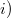$i)$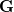$\mathbf{G}$ is also the support of a commutative group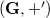$(\mathbf{G},+')$ such that$(\mathbf{G},+)$ and$(\mathbf{G},+')$ have the same cyclic cosets [cosets of order$p$].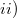$ii)$ the operation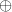$\oplus$ defined on$\mathbf{G}$ by putting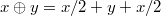$x \oplus y = x/2 + y + x/2$, gives$\mathbf{G}$ a structure of commutative group.\end

DOI Code: 10.1285/i15900932v30n2p149

Keywords: nilpotent groups; group partition

Full Text: PDF